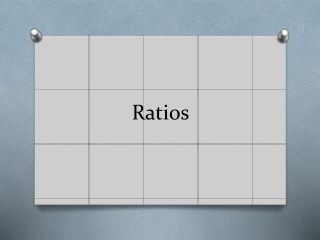DownloadDownload PresentationRatios

# Ratios

Télécharger la présentation## Ratios

- - - - - - - - - - - - - - - - - - - - - - - - - - - E N D - - - - - - - - - - - - - - - - - - - - - - - - - - -
##### Presentation Transcript

1. Ratios

2. What does a ratio do? • A ratio compares values. • It tells us how much of one thing there is compared to another thing. • There are 3 orange squares to 1 yellow square

3. How to show a ratio • Ratios can be shown in different ways: • Using the : to separate the values 3:1 • You can also use the word to 3 to 1

4. Ratios can be increased (scaled up) • We can multiply ratios in order to scale them up. • What happens if we multiply or scale up 3:1 by 2? 3:1 • Here the ratio is also 3 orange squares to 1 yellow square, even though there are more squares. 2 6

5. Using Ratios 4 : 5 x2 x2 8 : 10

6. Football Example: • A quarterback throws the ball 10 times in the first quarter of the game and 6 of his passes are caught. • If he has the same results in the remaining three quarters of the football game what are his final numbers?

8. Part-to-Part Ratios • Part-to-Part: • The ratio of boy to girl puppies is 2:3 • The ratio of girl to boy puppies is 3:2

9. Part-to-Whole Ratios • Part-to-Whole: • The ratio of boys to all puppies is 2:5 • The ratio of girls to all puppies is 3:5

10. Singer Example

11. Singer Ratios • The ratio of Katy Perry to Justin Bieber is 1:2 • The ratio of Justin Bieber to Katy Perry is 2:1 • The ratio of Katy Perry to all singers is 1:3 • The ratio of Justin Bieber to all singers is 2:3

12. Scaling • You can use ratios to scale drawings up or down (by multiplying or dividing).

13. Lobo Louie Example • The height to width ratio of Lobo Louie’s head is 2:3 • So for every 2 feet of height there should be 3 feet of width. • But if we wanted to use inches instead we would scale up by 12. • Then are ratio would become 24:36. • Lobo Louie has a big head!

14. Business Example • Let’s start a lemonade business! • We know that it takes 2 sugar packets for every 5 lemons to make 1 cup of lemonade. • How many sugar packets and lemons do we need to serve 20 customers?

16. Proportions

17. What does a proportion do? • A proportion says that two ratios (or fractions) are equal. 1-out-of-3is equal to 2-out-of-6 The ratios are the same, so they are in proportion.

18. How to show a Proportion • Proportions can be shown in different ways: • Fraction form • Word form1 out of 2

19. Using Proportions • When things are "in proportion" then their relative sizes are the same. • Here you can see that the ratios of head length to body length are the same in both drawings. • So they are proportional. (Both fractions can reduce to ½.)

20. Example: We want to draw the dog's head, and we need to know how long it should be: Write the proportion with the help of the 1/2 ratio from above:

21. Answer: Now we solve it mathematically: So you should draw the head 21 long.

22. How tall is the tree? Alex tried using a ladder, tape measure, ropes and various other things, but still couldn't work out how tall the tree was.

23. How tall is the tree? Alex has a clever idea: proportions! He measures a stick and its shadow (in meters), and also the shadow of the tree, and this is what he gets:

24. How tall is the tree? Alex writes the "Height to Length" ratio for both objects:

25. How tall is the tree? To solve, we multiply both sides by 2.9: Answer: the tree is 5.4 m tall. And he didn't even need a ladder!

26. Example Maggie and her little sister Minnie are in proportion.What is Minnie's height?

27. What is Minnie’s height?

28. Health Example If the records at the Humane Society show that they have 9 cats for every 10 dogs. • The ratio of cats to dogs can be written: 9 to 10 or 9:10.

29. Health Example If the records at the Humane Society show that they have a total of 40 dogs The total number of catsat the Humane Society can be found by using a proportion: Total # of cats =

30. Health Example About 4 in every 5 puppies in Albuquerque are taken to Animal Humane to be vaccinated. If we were all puppies in this classroom, how many of us would be vaccinated at Animal Humane?